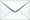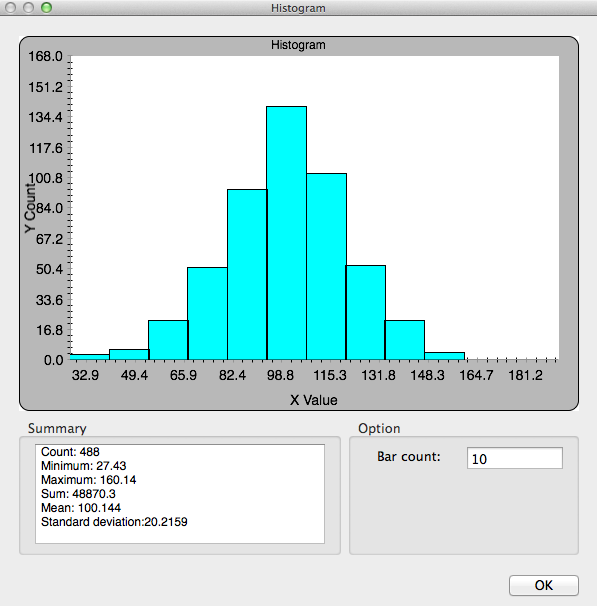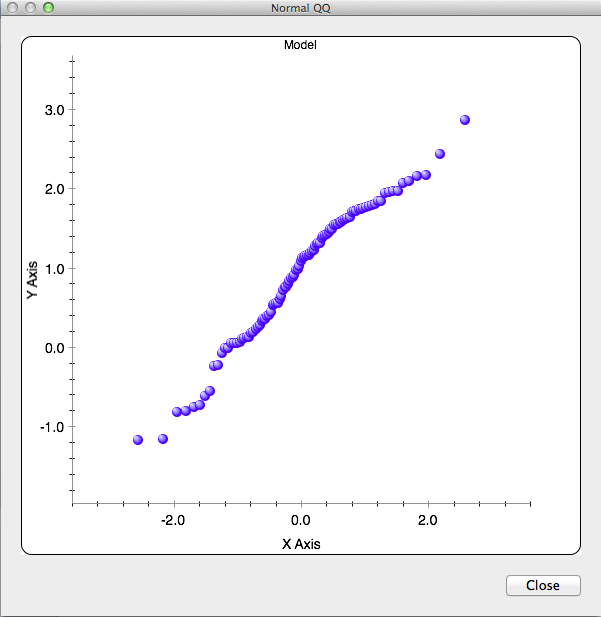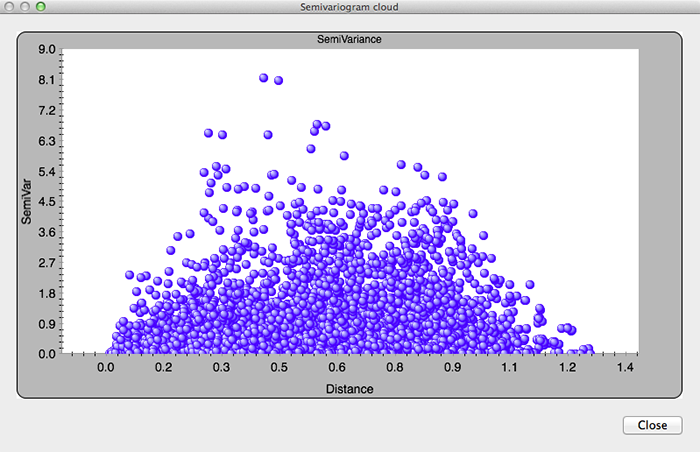Product Search

Dear customers:

take action as soon as possible.tyevolution@yahoo.com

EC: Point Layer__Interpolate to

Views:730times

Statistics

1. Histogram

The Histogram tool provides a univariate (one-variable) description of your data. The tool dialog box displays

the frequency distribution for the dataset of interest and calculates summary statistics.

The frequency distribution is a bar graph that displays how often observed values fall within certain intervals or

classes. You can specify the number of classes of equal width that are used in the histogram. The relative

proportion of data that falls in each class is represented by the height of each bar.2. Normal QQ Plot

Points on the Normal QQ plot provide an indication of univariate normality of the dataset. If the data is normally

distributed, the points will fall on the 45-degree reference line. If the data is not normally distributed, the points

will deviate from the reference line.

In the diagram below, the quantile values of the standard normal distribution are plotted on the x-axis in the

Normal QQ plot, and the corresponding quantile values of the dataset are plotted on the y-axis. You can see

that the points fall close to the 45-degree reference line.3. Semivariogram cloud